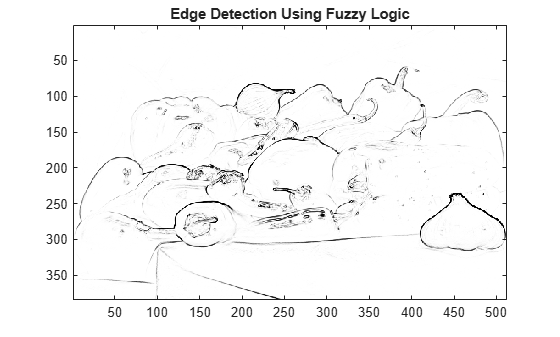# Fuzzy Logic Image Processing

This example shows how to use fuzzy logic for image processing. Specifically, this example shows how to detect edges in an image.

An edge is a boundary between two uniform regions. You can detect an edge by comparing the intensity of neighboring pixels. However, because uniform regions are not crisply defined, small intensity differences between two neighboring pixels do not always represent an edge. Instead, the intensity difference might represent a shading effect.

The fuzzy logic approach for image processing allows you to use membership functions to define the degree to which a pixel belongs to an edge or a uniform region.

### Import RGB Image and Convert to Grayscale

Import the image.

`Irgb = imread('peppers.png');`

`Irgb` is a 384 x 512 x 3 `uint8` array. The three channels of `Irgb` (third array dimension) represent the red, green, and blue intensities of the image.

Convert `Irgb` to grayscale so that you can work with a 2-D array instead of a 3-D array. To do so, use the `rgb2gray` function.

```Igray = rgb2gray(Irgb); figure image(Igray,'CDataMapping','scaled') colormap('gray') title('Input Image in Grayscale')```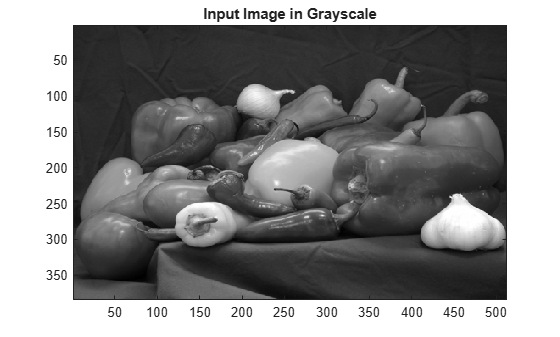### Convert Image to Double-Precision Data

The `evalfis` function for evaluating fuzzy inference systems supports only single-precision and double-precision data. Therefore, convert `Igray` to a `double` array using the `im2double` function.

`I = im2double(Igray);`

The fuzzy logic edge-detection algorithm for this example relies on the image gradient to locate breaks in uniform regions. Calculate the image gradient along the x-axis and y-axis.

`Gx` and `Gy` are simple gradient filters. To obtain a matrix containing the x-axis gradients of `I`, you convolve `I` with `Gx` using the `conv2` function. The gradient values are in the [-1 1] range. Similarly, to obtain the y-axis gradients of `I`, convolve `I` with `Gy`.

```Gx = [-1 1]; Gy = Gx'; Ix = conv2(I,Gx,'same'); Iy = conv2(I,Gy,'same');```

```figure image(Ix,'CDataMapping','scaled') colormap('gray') title('Ix')``````figure image(Iy,'CDataMapping','scaled') colormap('gray') title('Iy')```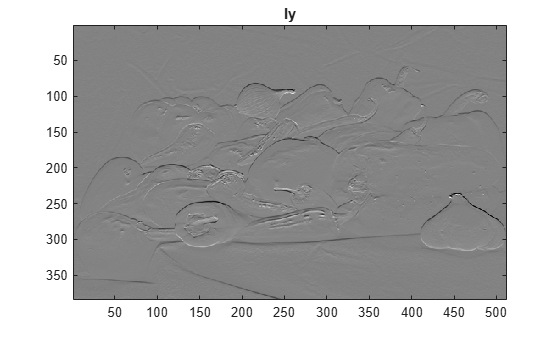You can use other filters to obtain the image gradients, such as the Sobel operator or the Prewitt operator. For information about how you can filter an image using convolution, see What Is Image Filtering in the Spatial Domain? (Image Processing Toolbox)

Alternatively, if you have the Image Processing Toolbox software, you can use the `imfilter` (Image Processing Toolbox), `imgradientxy` (Image Processing Toolbox), or `imgradient` (Image Processing Toolbox) functions to obtain the image gradients.

### Define Fuzzy Inference System (FIS) for Edge Detection

Create a fuzzy inference system (FIS) for edge detection, `edgeFIS`.

`edgeFIS = mamfis('Name','edgeDetection');`

Specify the image gradients, `Ix` and `Iy`, as the inputs of `edgeFIS`.

```edgeFIS = addInput(edgeFIS,[-1 1],'Name','Ix'); edgeFIS = addInput(edgeFIS,[-1 1],'Name','Iy');```

Specify a zero-mean Gaussian membership function for each input. If the gradient value for a pixel is `0`, then it belongs to the zero membership function with a degree of `1`.

```sx = 0.1; sy = 0.1; edgeFIS = addMF(edgeFIS,'Ix','gaussmf',[sx 0],'Name','zero'); edgeFIS = addMF(edgeFIS,'Iy','gaussmf',[sy 0],'Name','zero');```

`sx` and `sy` specify the standard deviation for the zero membership function for the `Ix` and `Iy` inputs. To adjust the edge detector performance, you can change the values of `sx` and `sy`. Increasing the values makes the algorithm less sensitive to the edges in the image and decreases the intensity of the detected edges.

Specify the intensity of the edge-detected image as an output of `edgeFIS`.

`edgeFIS = addOutput(edgeFIS,[0 1],'Name','Iout');`

Specify the triangular membership functions, white and black, for `Iout`.

```wa = 0.1; wb = 1; wc = 1; ba = 0; bb = 0; bc = 0.7; edgeFIS = addMF(edgeFIS,'Iout','trimf',[wa wb wc],'Name','white'); edgeFIS = addMF(edgeFIS,'Iout','trimf',[ba bb bc],'Name','black');```

As you can with `sx` and `sy`, you can change the values of `wa`, `wb`, `wc`, `ba`, `bb`, and `bc` to adjust the edge detector performance. The triplets specify the start, peak, and end of the triangles of the membership functions. These parameters influence the intensity of the detected edges.

Plot the membership functions of the inputs and outputs of `edgeFIS`.

```figure subplot(2,2,1) plotmf(edgeFIS,'input',1) title('Ix') subplot(2,2,2) plotmf(edgeFIS,'input',2) title('Iy') subplot(2,2,[3 4]) plotmf(edgeFIS,'output',1) title('Iout')```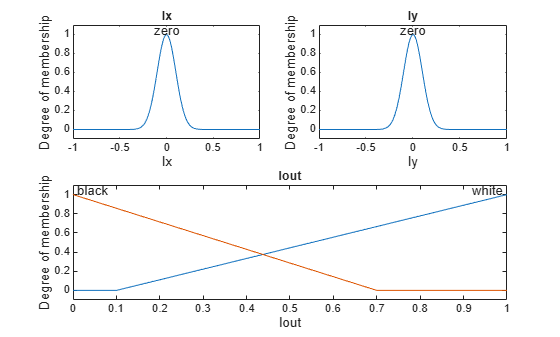### Specify FIS Rules

Add rules to make a pixel white if it belongs to a uniform region and black otherwise. A pixel is in a uniform region when the image gradient is zero in both directions. If either direction has a nonzero gradient, then the pixel is on an edge.

```r1 = "If Ix is zero and Iy is zero then Iout is white"; r2 = "If Ix is not zero or Iy is not zero then Iout is black"; edgeFIS = addRule(edgeFIS,[r1 r2]); edgeFIS.Rules```
```ans = 1x2 fisrule array with properties: Description Antecedent Consequent Weight Connection Details: Description _______________________________________ 1 "Ix==zero & Iy==zero => Iout=white (1)" 2 "Ix~=zero | Iy~=zero => Iout=black (1)" ```

### Evaluate FIS

Evaluate the output of the edge detector for each row of pixels in `I` using corresponding rows of `Ix` and `Iy` as inputs.

```Ieval = zeros(size(I)); for ii = 1:size(I,1) Ieval(ii,:) = evalfis(edgeFIS,[(Ix(ii,:));(Iy(ii,:))]'); end```

### Plot Results

Plot the original grayscale image.

```figure image(I,'CDataMapping','scaled') colormap('gray') title('Original Grayscale Image')```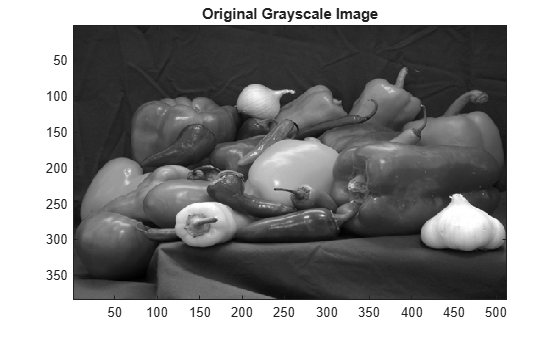Plot the detected edges.

```figure image(Ieval,'CDataMapping','scaled') colormap('gray') title('Edge Detection Using Fuzzy Logic')```